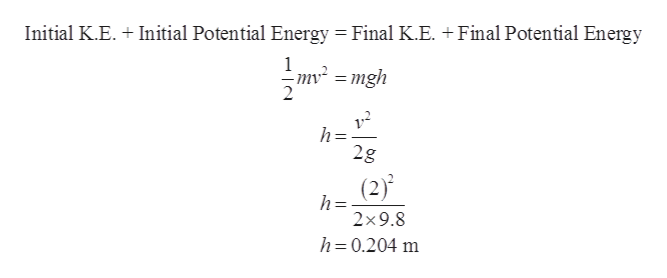# A pendulum consists of a small object hanging from the ceiling at the end of a string of negligible mass. The string has a length of 0.75 m. With the string hanging vertically, the object is given an initial velocity of 2.0 m/s parallel to the ground and swings upward on a circular arc. Eventually, the object comes to a momentary halt at a point where the string makes an angle θ with its initial vertical orientation and then swings back downward. Find the angle θ.

Question
70 views

A pendulum consists of a small object hanging from the ceiling at the end of a string of negligible mass. The string has a length of 0.75 m. With the string hanging vertically, the object is given an initial velocity of 2.0 m/s parallel to the ground and swings upward on a circular arc. Eventually, the object comes to a momentary halt at a point where the string makes an angle θ with its initial vertical orientation and then swings back downward. Find the angle θ.

check_circle

Step 1

Given,

Step 2

Diagram,

Step 3

Let the final speed of the object is zero at point A and take D point ...help_outlineImage TranscriptioncloseInitial K.E. Initial Potential Energy Final K.E. +Final Potential Energy 1 mv2=mgh h= 2g (2) h= 2*9.8 h 0.204 m fullscreen

### Want to see the full answer?

See Solution

#### Want to see this answer and more?

Solutions are written by subject experts who are available 24/7. Questions are typically answered within 1 hour.*

See Solution
*Response times may vary by subject and question.
Tagged in

### Other